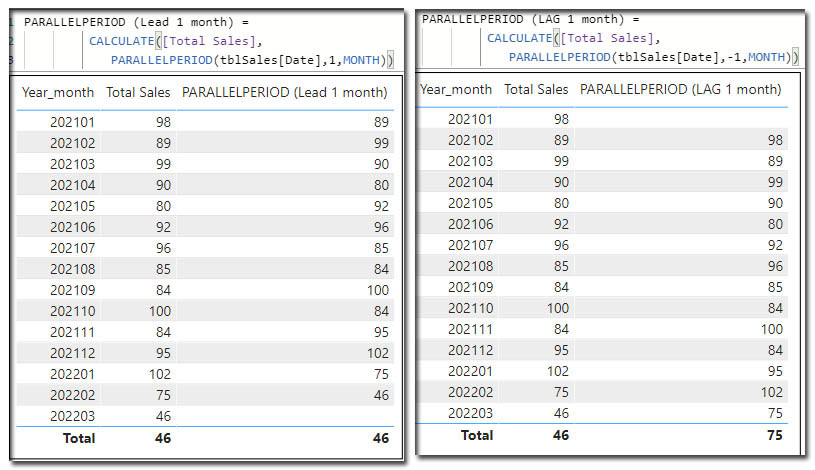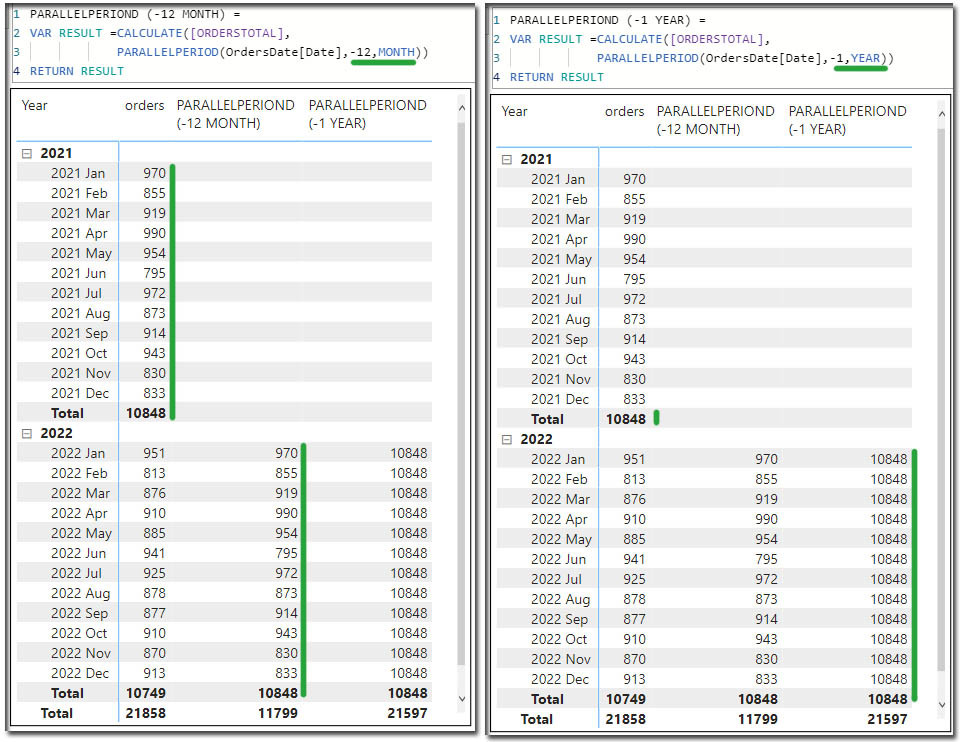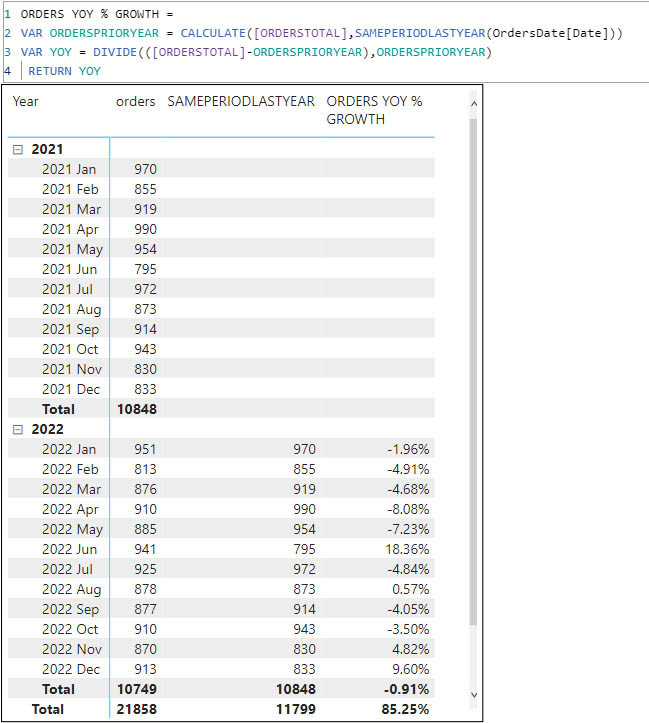Search
• Marek Vavrovic

# Time intelligence functions, part 03

Updated: Sep 27

## FIRSTDATE, LASTDATE

FIRSTDATE returns first non blank date LASTDATE returns last non blank date.

Example

I want to calculate first 15 days of the sale.

FIRST 15 DAYS SALE =

VAR _START = DATE (2021, 01, 01)

VAR _END = DATE (2021, 01, 15)

VAR _RESULT =

CALCULATE (SUM ( tblSales[Sales] ),

FILTER (tblSales,

(

FIRSTDATE ( tblSales[Date] ) >= _START

&& LASTDATE ( tblSales[Date] ) <= _END

)

))

RETURN _RESULTNOTE: You can use this measure, to calculate the running total for the first 15 days

FIRST 15 DAYS CUM_SALE =

VAR Period =

DATESBETWEEN ( tblSales[Date], DATE (2021, 01, 01), DATE (2021, 1, 15))

VAR _RESULT =CALCULATE (SUM ( tblSales[Sales] ),

DATESYTD ( tblSales[Date], "2021-01-15"), Period)

RETURN _RESULTExample

step 1 > create 2 measure, FistDateSale & LastDateSale

_FirstDateSale = CALCULATE([Total Sales],FIRSTDATE(tblSales[Date]))

_LastDateSale = CALCULATE([Total Sales],LASTDATE(tblSales[Date]))

measure for the Total Sales is as follow:

Total Sales = CALCULATE(SUM(tblSales[Sales]),DATESMTD(tblSales[Date]))

This measure is calculating the running total in the month.

You can use the measures in the summarized table. This table is grouped be Year_month column.## FIRSTNONBLANK, LASTNONBLANK

FIRSTNONBLANK: returns the first value in the column for which the expression has a non blank value.

LASTNONBLANK: returns the last value in the column for which the expression has a non blank value.

syntax:

FIRSTNONBLANK(<column>,<expression>)

LASTNONBLANK(<column>,<expression>)

Example

I want to retrieve specified date from the table.Example

I want to create a measure which will contain the sum of occurrences of values in Column A based on the values in Column B.

NUMBER OF OCCURRENCES =

VAR COL_B =

FIRSTNONBLANK ( LETTERS[Column B], "")

VAR RESULT =

CALCULATE (COUNTROWS ( LETTERS ), ALL (), LETTERS[Column A ] = COL_B)

RETURN

IF (ISBLANK (RESULT), 0, RESULT)## NEXTDAY,NEXTMONTH,NEXTQUARTER,NEXTYEAR

NEXTDAY () is similar to DATEADD()

syntax

NEXTDAY(<dates>)

_NextDay = CALCULATE([Total Sales],NEXTDAY(tblSales[Date]))NEXTMONTH

Returns a next month

NEXTMONTH(<dates>)NEXTYEAR

Returns a table that contains a column of all dates in the next year, based on the first date in the dates column, in the current context.

NEXTYEAR(<dates>[,<year_end_date>])

NEXTYEAR() function returned the sum for the next year 2022 (102+75+46 = 223)_NEXTYEAR =

CALCULATE(SUM(tblSales[Sales]), NEXTYEAR(tblSales[Date],"2021-01-31"))

[,<year_end_date>] is an optional argument, you can use it for the fiscal year calculation.## PARALLELPERIOD

Returns a parallel period of dates by the given set of dates and a specified interval.

PARALLELPERIOD returns a full period shifted in time.

PARALLELPERIOD(<dates>,<number_of_intervals>,<interval>)

<interval> month, quarter, yearExample

This picture demonstrate a difference between two different entries in the PARALLELPERIOD () function. <-12,MONTH> will shift the dates, <-1,YAER> returns a grand total for each row.## SAMEPERIODLASTYEAR

Returns a set of dates in the current selection from the previous year.

syntax

SAMEPERIODLASTYEAR(<dates>)

This function is similar to DATEADD(OrdersDate[Date],-1,YEAR)) or PARALLELPERIOD(OrdersDate[Date],-12,MONTH)).

ExampleExample

Calculating Year over year percentage growth

ORDERS YOY % GROWTH =

VAR ORDERSPRIORYEAR =

CALCULATE ( [ORDERSTOTAL], SAMEPERIODLASTYEAR ( OrdersDate[Date] ))

VAR YOY =

DIVIDE ( ( [ORDERSTOTAL] - ORDERSPRIORYEAR), ORDERSPRIORYEAR)

RETURN YOY## Recent Posts

See All

#### Index

Information functions Filter functions Table manipulation functions Grouping and summarizing Time intelligence functions OPENINGBALANCEYEAR OPENINGBALANCEYEAR(<Expression>,<Dates> [,<Filter>][,<YearEn